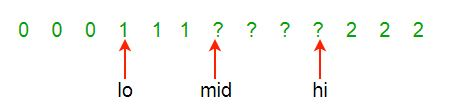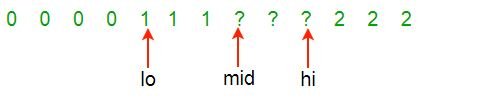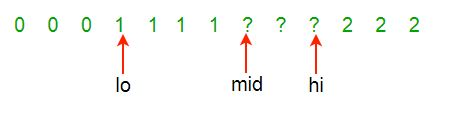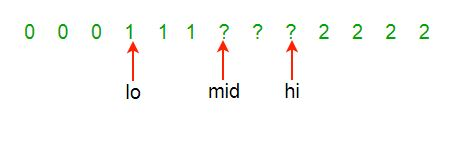# Sort an array of 0s, 1s and 2s | Dutch National Flag problem

• Difficulty Level : Medium
• Last Updated : 28 Jun, 2022

Given an array A[] consisting only 0s, 1s and 2s. The task is to write a function that sorts the given array. The functions should put all 0s first, then all 1s and all 2s in last.

This problem is also the same as the famous “Dutch National Flag problem”. The problem was proposed by Edsger Dijkstra. The problem is as follows:

Given N balls of colour red, white or blue arranged in a line in random order. You have to arrange all the balls such that the balls with the same colours are adjacent with the order of the balls, with the order of the colours being red, white and blue (i.e., all red coloured balls come first then the white coloured balls and then the blue coloured balls).

Examples:

Input: {0, 1, 2, 0, 1, 2}
Output: {0, 0, 1, 1, 2, 2}

Input: {0, 1, 1, 0, 1, 2, 1, 2, 0, 0, 0, 1}
Output: {0, 0, 0, 0, 0, 1, 1, 1, 1, 1, 2, 2}

A simple solution is discussed in this(Sort an array of 0s, 1s and 2s (Simple Counting)) post.
Method 1

• Approach: The problem is similar to our old post “Segregate 0s and 1s in an array”.
The problem was posed with three colours, here `0′, `1′ and `2′. The array is divided into four sections:
1. a[1..Lo-1] zeroes (red)
2. a[Lo..Mid-1] ones (white)
3. a[Mid..Hi] unknown
4. a[Hi+1..N] twos (blue)
5. If the ith element is 0 then swap the element to the low range, thus shrinking the unknown range.
6. Similarly, if the element is 1 then keep it as it is but shrink the unknown range.
7. If the element is 2 then swap it with an element in high range.
• Algorithm:
1. Keep three indices low = 1, mid = 1 and high = N and there are four ranges, 1 to low (the range containing 0), low to mid (the range containing 1), mid to high (the range containing unknown elements) and high to N (the range containing 2).
2. Traverse the array from start to end and mid is less than high. (Loop counter is i)
3. If the element is 0 then swap the element with the element at index low and update low = low + 1 and mid = mid + 1
4. If the element is 1 then update mid = mid + 1
5. If the element is 2 then swap the element with the element at index high and update high = high – 1 and update i = i – 1. As the swapped element is not processed
6. Print the array.
• Dry Run:
Part way through the process, some red, white and blue elements are known and are in the “right” place. The section of unknown elements, a[Mid..Hi], is shrunk by examining a[Mid]:Examine a[Mid]. There are three possibilities:
a[Mid] is (0) red, (1) white or (2) blue.
Case (0) a[Mid] is red, swap a[Lo] and a[Mid]; Lo++; Mid++Case (1) a[Mid] is white, Mid++Case (2) a[Mid] is blue, swap a[Mid] and a[Hi]; Hi–Continue until Mid>Hi.

• Implementation:

## C++

 `// C++ program to sort an array``// with 0, 1 and 2 in a single pass``#include ``using` `namespace` `std;`` ` `// Function to sort the input array,``// the array is assumed``// to have values in {0, 1, 2}``void` `sort012(``int` `a[], ``int` `arr_size)``{``    ``int` `lo = 0;``    ``int` `hi = arr_size - 1;``    ``int` `mid = 0;`` ` `    ``// Iterate till all the elements``    ``// are sorted``    ``while` `(mid <= hi) {``        ``switch` `(a[mid]) {`` ` `        ``// If the element is 0``        ``case` `0:``            ``swap(a[lo++], a[mid++]);``            ``break``;`` ` `        ``// If the element is 1 .``        ``case` `1:``            ``mid++;``            ``break``;`` ` `        ``// If the element is 2``        ``case` `2:``            ``swap(a[mid], a[hi--]);``            ``break``;``        ``}``    ``}``}`` ` `// Function to print array arr[]``void` `printArray(``int` `arr[], ``int` `arr_size)``{``    ``// Iterate and print every element``    ``for` `(``int` `i = 0; i < arr_size; i++)``        ``cout << arr[i] << ``" "``;``}`` ` `// Driver Code``int` `main()``{``    ``int` `arr[] = { 0, 1, 1, 0, 1, 2, 1, 2, 0, 0, 0, 1 };``    ``int` `n = ``sizeof``(arr) / ``sizeof``(arr);`` ` `    ``sort012(arr, n);`` ` `    ``printArray(arr, n);`` ` `    ``return` `0;``}`` ` `// This code is contributed by Shivi_Aggarwal`

## C

 `// C program to sort an array with 0, 1 and 2``// in a single pass``#include `` ` `/* Function to swap *a and *b */``void` `swap(``int``* a, ``int``* b);`` ` `// Sort the input array, the array is assumed to``// have values in {0, 1, 2}``void` `sort012(``int` `a[], ``int` `arr_size)``{``    ``int` `lo = 0;``    ``int` `hi = arr_size - 1;``    ``int` `mid = 0;`` ` `    ``while` `(mid <= hi) {``        ``switch` `(a[mid]) {``        ``case` `0:``            ``swap(&a[lo++], &a[mid++]);``            ``break``;``        ``case` `1:``            ``mid++;``            ``break``;``        ``case` `2:``            ``swap(&a[mid], &a[hi--]);``            ``break``;``        ``}``    ``}``}`` ` `/* UTILITY FUNCTIONS */``void` `swap(``int``* a, ``int``* b)``{``    ``int` `temp = *a;``    ``*a = *b;``    ``*b = temp;``}`` ` `/* Utility function to print array arr[] */``void` `printArray(``int` `arr[], ``int` `arr_size)``{``    ``int` `i;``    ``for` `(i = 0; i < arr_size; i++)``        ``printf``(``"%d "``, arr[i]);``    ``printf``(``"n"``);``}`` ` `/* driver program to test */``int` `main()``{``    ``int` `arr[] = { 0, 1, 1, 0, 1, 2, 1, 2, 0, 0, 0, 1 };``    ``int` `arr_size = ``sizeof``(arr) / ``sizeof``(arr);``    ``int` `i;`` ` `    ``sort012(arr, arr_size);`` ` `    ``printArray(arr, arr_size);`` ` `    ``getchar``();``    ``return` `0;``}`

## Java

 `// Java program to sort an array of 0, 1 and 2``import` `java.io.*;`` ` `class` `countzot {`` ` `    ``// Sort the input array, the array is assumed to``    ``// have values in {0, 1, 2}``    ``static` `void` `sort012(``int` `a[], ``int` `arr_size)``    ``{``        ``int` `lo = ``0``;``        ``int` `hi = arr_size - ``1``;``        ``int` `mid = ``0``, temp = ``0``;``        ``while` `(mid <= hi) {``            ``switch` `(a[mid]) {``            ``case` `0``: {``                ``temp = a[lo];``                ``a[lo] = a[mid];``                ``a[mid] = temp;``                ``lo++;``                ``mid++;``                ``break``;``            ``}``            ``case` `1``:``                ``mid++;``                ``break``;``            ``case` `2``: {``                ``temp = a[mid];``                ``a[mid] = a[hi];``                ``a[hi] = temp;``                ``hi--;``                ``break``;``            ``}``            ``}``        ``}``    ``}`` ` `    ``/* Utility function to print array arr[] */``    ``static` `void` `printArray(``int` `arr[], ``int` `arr_size)``    ``{``        ``int` `i;``        ``for` `(i = ``0``; i < arr_size; i++)``            ``System.out.print(arr[i] + ``" "``);``        ``System.out.println(``""``);``    ``}`` ` `    ``/*Driver function to check for above functions*/``    ``public` `static` `void` `main(String[] args)``    ``{``        ``int` `arr[] = { ``0``, ``1``, ``1``, ``0``, ``1``, ``2``, ``1``, ``2``, ``0``, ``0``, ``0``, ``1` `};``        ``int` `arr_size = arr.length;``        ``sort012(arr, arr_size);``        ``printArray(arr, arr_size);``    ``}``}``/*This code is contributed by Devesh Agrawal*/`

## Python3

 `# Python program to sort an array with ``# 0, 1 and 2 in a single pass`` ` `# Function to sort array``def` `sort012( a, arr_size):``    ``lo ``=` `0``    ``hi ``=` `arr_size ``-` `1``    ``mid ``=` `0``    ``while` `mid <``=` `hi:``        ``if` `a[mid] ``=``=` `0``:``            ``a[lo], a[mid] ``=` `a[mid], a[lo]``            ``lo ``=` `lo ``+` `1``            ``mid ``=` `mid ``+` `1``        ``elif` `a[mid] ``=``=` `1``:``            ``mid ``=` `mid ``+` `1``        ``else``:``            ``a[mid], a[hi] ``=` `a[hi], a[mid] ``            ``hi ``=` `hi ``-` `1``    ``return` `a``     ` `# Function to print array``def` `printArray( a):``    ``for` `k ``in` `a:``        ``print``(k,end``=``' '``)``   ` `# Driver Program``arr ``=` `[``0``, ``1``, ``1``, ``0``, ``1``, ``2``, ``1``, ``2``, ``0``, ``0``, ``0``, ``1``]``arr_size ``=` `len``(arr)``arr ``=` `sort012( arr, arr_size)``printArray(arr)`` ` `# Contributed by Harshit Agrawal`

## C#

 `// C# program to sort an``// array of 0, 1 and 2``using` `System;`` ` `class` `GFG {``    ``// Sort the input array, the array is assumed to``    ``// have values in {0, 1, 2}``    ``static` `void` `sort012(``int``[] a, ``int` `arr_size)``    ``{``        ``int` `lo = 0;``        ``int` `hi = arr_size - 1;``        ``int` `mid = 0, temp = 0;`` ` `        ``while` `(mid <= hi) {``            ``switch` `(a[mid]) {``            ``case` `0: {``                ``temp = a[lo];``                ``a[lo] = a[mid];``                ``a[mid] = temp;``                ``lo++;``                ``mid++;``                ``break``;``            ``}``            ``case` `1:``                ``mid++;``                ``break``;``            ``case` `2: {``                ``temp = a[mid];``                ``a[mid] = a[hi];``                ``a[hi] = temp;``                ``hi--;``                ``break``;``            ``}``            ``}``        ``}``    ``}`` ` `    ``/* Utility function to print array arr[] */``    ``static` `void` `printArray(``int``[] arr, ``int` `arr_size)``    ``{``        ``int` `i;`` ` `        ``for` `(i = 0; i < arr_size; i++)``            ``Console.Write(arr[i] + ``" "``);``        ``Console.WriteLine(``""``);``    ``}`` ` `    ``/*Driver function to check for above functions*/``    ``public` `static` `void` `Main()``    ``{``        ``int``[] arr = { 0, 1, 1, 0, 1, 2, 1, 2, 0, 0, 0, 1 };``        ``int` `arr_size = arr.Length;``        ``sort012(arr, arr_size);`` ` `        ``printArray(arr, arr_size);``    ``}``}`` ` `// This code is contributed by Sam007`

## PHP

 ``

## Javascript

 ``

Output

`0 0 0 0 0 1 1 1 1 1 2 2 `

Complexity Analysis:

• Time Complexity: O(n).
Only one traversal of the array is needed.
• Space Complexity: O(1).
No extra space is required.

Approach: Count the number of 0s, 1s and 2s in the given array. Then store all the 0s in the beginning followed by all the 1s then all the 2s.

• Algorithm:
1. Keep three counter c0 to count 0s, c1 to count 1s and c2 to count 2s
2. Traverse through the array and increase the count of c0 if the element is 0,increase the count of c1 if the element is 1 and increase the count of c2 if the element is 2
3. Now again traverse the array and replace first c0 elements with 0, next c1 elements with 1 and next c2 elements with 2.
• Implementation:

## C++

 `// C++ implementation of the approach``#include ``using` `namespace` `std;`` ` `// Utility function to print the contents of an array``void` `printArr(``int` `arr[], ``int` `n)``{``    ``for` `(``int` `i = 0; i < n; i++)``        ``cout << arr[i] << ``" "``;``}`` ` `// Function to sort the array of 0s, 1s and 2s``void` `sortArr(``int` `arr[], ``int` `n)``{``    ``int` `i, cnt0 = 0, cnt1 = 0, cnt2 = 0;`` ` `    ``// Count the number of 0s, 1s and 2s in the array``    ``for` `(i = 0; i < n; i++) {``        ``switch` `(arr[i]) {``        ``case` `0:``            ``cnt0++;``            ``break``;``        ``case` `1:``            ``cnt1++;``            ``break``;``        ``case` `2:``            ``cnt2++;``            ``break``;``        ``}``    ``}`` ` `    ``// Update the array``    ``i = 0;`` ` `    ``// Store all the 0s in the beginning``    ``while` `(cnt0 > 0) {``        ``arr[i++] = 0;``        ``cnt0--;``    ``}`` ` `    ``// Then all the 1s``    ``while` `(cnt1 > 0) {``        ``arr[i++] = 1;``        ``cnt1--;``    ``}`` ` `    ``// Finally all the 2s``    ``while` `(cnt2 > 0) {``        ``arr[i++] = 2;``        ``cnt2--;``    ``}`` ` `    ``// Print the sorted array``    ``printArr(arr, n);``}`` ` `// Driver code``int` `main()``{``    ``int` `arr[] = { 0, 1, 1, 0, 1, 2, 1, 2, 0, 0, 0, 1 };``    ``int` `n = ``sizeof``(arr) / ``sizeof``(``int``);`` ` `    ``sortArr(arr, n);`` ` `    ``return` `0;``}`

## Java

 `// Java implementation of the approach``import` `java.io.*;`` ` `class` `GFG {``    ``// Utility function to print the contents of an array``    ``static` `void` `printArr(``int` `arr[], ``int` `n)``    ``{``        ``for` `(``int` `i = ``0``; i < n; i++)``            ``System.out.print(arr[i] + ``" "``);``    ``}``     ` `    ``// Function to sort the array of 0s, 1s and 2s``    ``static` `void` `sortArr(``int` `arr[], ``int` `n)``    ``{``        ``int` `i, cnt0 = ``0``, cnt1 = ``0``, cnt2 = ``0``;``     ` `        ``// Count the number of 0s, 1s and 2s in the array``        ``for` `(i = ``0``; i < n; i++) {``            ``switch` `(arr[i]) {``            ``case` `0``:``                ``cnt0++;``                ``break``;``            ``case` `1``:``                ``cnt1++;``                ``break``;``            ``case` `2``:``                ``cnt2++;``                ``break``;``            ``}``        ``}``     ` `        ``// Update the array``        ``i = ``0``;``     ` `        ``// Store all the 0s in the beginning``        ``while` `(cnt0 > ``0``) {``            ``arr[i++] = ``0``;``            ``cnt0--;``        ``}``     ` `        ``// Then all the 1s``        ``while` `(cnt1 > ``0``) {``            ``arr[i++] = ``1``;``            ``cnt1--;``        ``}``     ` `        ``// Finally all the 2s``        ``while` `(cnt2 > ``0``) {``            ``arr[i++] = ``2``;``            ``cnt2--;``        ``}``     ` `        ``// Print the sorted array``        ``printArr(arr, n);``    ``}``     ` `    ``// Driver code``    ``public` `static` `void` `main(String[] args)``    ``{``        ``int` `arr[] = { ``0``, ``1``, ``1``, ``0``, ``1``, ``2``, ``1``, ``2``, ``0``, ``0``, ``0``, ``1` `};``        ``int` `n = arr.length;``        ``sortArr(arr, n);``    ``}``}`` ` `// This code is contributed by shubhamsingh10`

## Python3

 `# Python implementation of the approach`` ` `# Utility function to print contents of an array``def` `printArr(arr, n):``    ``for` `i ``in` `range``(n):``        ``print``(arr[i],end``=``" "``)`` ` ` ` `# Function to sort the array of 0s, 1s and 2s``def` `sortArr(arr, n):``    ``cnt0 ``=` `0``    ``cnt1 ``=` `0``    ``cnt2 ``=` `0``     ` `    ``# Count the number of 0s, 1s and 2s in the array``    ``for` `i ``in` `range``(n):``        ``if` `arr[i] ``=``=` `0``:``            ``cnt0``+``=``1``         ` `        ``elif` `arr[i] ``=``=` `1``:``            ``cnt1``+``=``1``             ` `        ``elif` `arr[i] ``=``=` `2``:``            ``cnt2``+``=``1``     ` `    ``# Update the array``    ``i ``=` `0``     ` `    ``# Store all the 0s in the beginning``    ``while` `(cnt0 > ``0``):``        ``arr[i] ``=` `0``        ``i``+``=``1``        ``cnt0``-``=``1``     ` `    ``# Then all the 1s``    ``while` `(cnt1 > ``0``):``        ``arr[i] ``=` `1``        ``i``+``=``1``        ``cnt1``-``=``1``     ` `    ``# Finally all the 2s``    ``while` `(cnt2 > ``0``):``        ``arr[i] ``=` `2``        ``i``+``=``1``        ``cnt2``-``=``1``     ` `    ``# Print the sorted array``    ``printArr(arr, n)`` ` ` ` `# Driver code`` ` `arr ``=` `[``0``, ``1``, ``1``, ``0``, ``1``, ``2``, ``1``, ``2``, ``0``, ``0``, ``0``, ``1``]``n ``=` `len``(arr)`` ` `sortArr(arr, n)`` ` `#This code is contributed by shubhamsingh10`

## C#

 `// C# implementation of the approach``using` `System;``  ` `class` `GFG {``    ``// Utility function to print the contents of an array``    ``static` `void` `printArr(``int``[] arr, ``int` `n)``    ``{``        ``for` `(``int` `i = 0; i < n; i++)``            ``Console.Write(arr[i] + ``" "``);``    ``}``     ` `    ``// Function to sort the array of 0s, 1s and 2s``    ``static` `void` `sortArr(``int``[] arr, ``int` `n)``    ``{``        ``int` `i, cnt0 = 0, cnt1 = 0, cnt2 = 0;``     ` `        ``// Count the number of 0s, 1s and 2s in the array``        ``for` `(i = 0; i < n; i++) {``            ``switch` `(arr[i]) {``            ``case` `0:``                ``cnt0++;``                ``break``;``            ``case` `1:``                ``cnt1++;``                ``break``;``            ``case` `2:``                ``cnt2++;``                ``break``;``            ``}``        ``}``     ` `        ``// Update the array``        ``i = 0;``     ` `        ``// Store all the 0s in the beginning``        ``while` `(cnt0 > 0) {``            ``arr[i++] = 0;``            ``cnt0--;``        ``}``     ` `        ``// Then all the 1s``        ``while` `(cnt1 > 0) {``            ``arr[i++] = 1;``            ``cnt1--;``        ``}``     ` `        ``// Finally all the 2s``        ``while` `(cnt2 > 0) {``            ``arr[i++] = 2;``            ``cnt2--;``        ``}``     ` `        ``// Print the sorted array``        ``printArr(arr, n);``    ``}``     ` `    ``// Driver code``    ``public` `static` `void` `Main()``    ``{``        ``int``[] arr = { 0, 1, 1, 0, 1, 2, 1, 2, 0, 0, 0, 1 };``        ``int` `n = arr.Length;``     ` `        ``sortArr(arr, n);``    ``}``}`` ` `// This code is contributed by shubhamsingh10`

## Javascript

 ``

Output

`0 0 0 0 0 1 1 1 1 1 2 2 `

Complexity Analysis:

• Time Complexity: O(n).
Only non nested traversals of the array is needed.
• Space Complexity: O(1).
As no extra space is required.

My Personal Notes arrow_drop_up